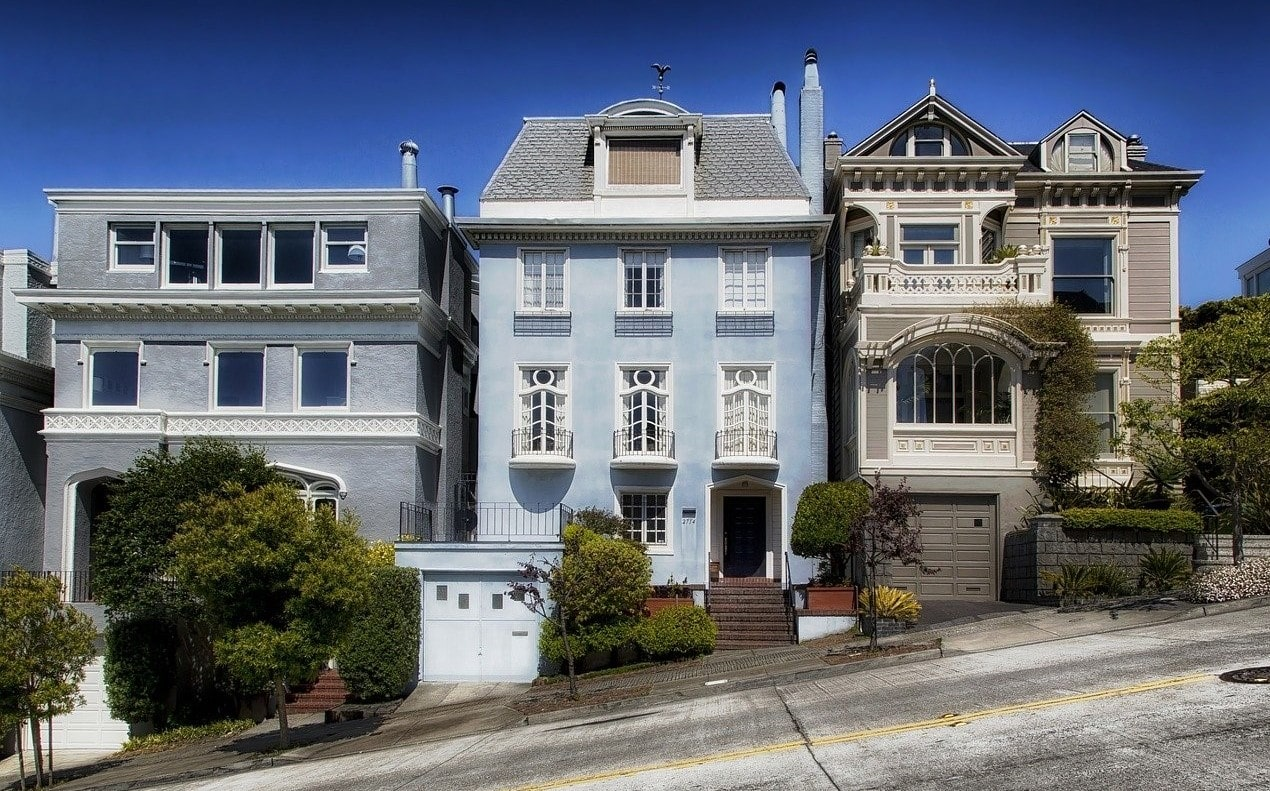No. The term "cap rate" gets used a whole lot in the real estate business and probably gets more play than it really deserves. What most people really want to know is what return a real estate investment will generate. That way, they can compare real estate investment returns to expected returns from other asset classes such as stocks, bonds or commodities.

The cap rate tells us only about the amount of expected net income in the first year of ownership. It is the ratio of year one income over the purchase price. By contrast, a return on investment calculation would take into consideration the net income generated each year throughout the entire holding period as well as the amount of proceeds at sale. The return could vary greatly depending upon the level and cost of any debt financing used to acquire the property.

The best way to calculate the expected return on investment is to make a projection of the expected cash flows during each year of the holding period and then perform DCF analysis. Investors can use the Internal Rate of Return (IRR) function in a financial calculator or Excel spreadsheet to run the computation.### Can you convert a cap rate into an investment return?

If investors know the cap rate for an asset, they can convert it into an expected investment return by adding the expected cash flow growth rate to the cap rate. The result shows the investment return assuming no debt financing is used to buy the property. Note that the cash flow growth rate will equal the rental growth rate only if rental income and operating expenses grow at the same rate. This short cut method only works if the going-in cap rate and the terminal cap rate are expected to be the same.

If an investor plans to use debt in the capital structure, knowing the unleveraged expected return can come in handy, since it can be used to perform DCF analysis. This would allow the investor to project leveraged investment returns using various levels of financing or determine the Net Present Value of the cash flows.

### Can you convert total return expectations into a cap rate?

Yes. If an investor can project the expected unleveraged return for a particular real estate investment, then it is possible to convert that return into a cap rate. The expected return should stem from both annual operating cash flow and appreciation. An investor can convert an expected return into a cap rate by subtracting the cash flow growth rate from the total return.### What methods are used to compute total expected returns?

There is no single best method to estimate total expected returns for real estate. Some of the most commonly used approaches are as follows:

This approach reveals what total returns should be using historical risk premiums to represent current return expectations. Investors can use the formula below to calculate an appropriate discount rate for an investment:

Total Return = Risk Free Rate + Real Estate Risk Premium

2. The Build-up Method:

The building-up method starts with the 10-year treasury yield and adds premiums for risk factors that add risk to the investment. Some common risk factors include:

• Deal Size Risk.
• Construction Quality Risk.
• Property Management Risk.
• Market Liquidity Risk. Investors can use the APV Location Ranking System to estimate the liquidity of a property in a given market.
3. Market Extraction Approach:

This entails estimating expected returns by observing cap rates at comparable properties and then converting them into total returns by adding the expected long-term growth rate in cash flow to the cap rate. Local real estate appraisers, real estate brokers or service providers are usually the best source for finding observed cap rates at comparable properties.

4. Survey Approach:

This involves using surveys to ask real estate investors what their return expectations are for specific types of real estate investments.

### How can you find the expected return for a particular piece of real estate?

Use the approaches above to figure it out. Alternatively, you can enter the property address, physical characteristics and income and expense projections into the cap rate calculator at apartmentpropertyvaluation.com. The tool provides cap rates for properties throughout the United States.## Find Cap Rates for Rental Housing

It takes just four minutes